# 如何判别凹多边形

## 问题描述

《计算机图形学》3.15.1 多边形分类 这一小节里有这样的描述：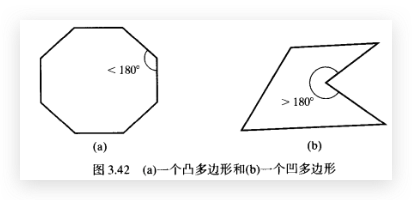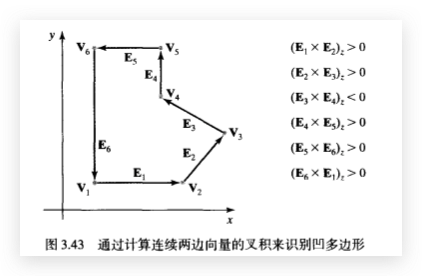## 理解边向量叉积方法

### 1. 移动边向量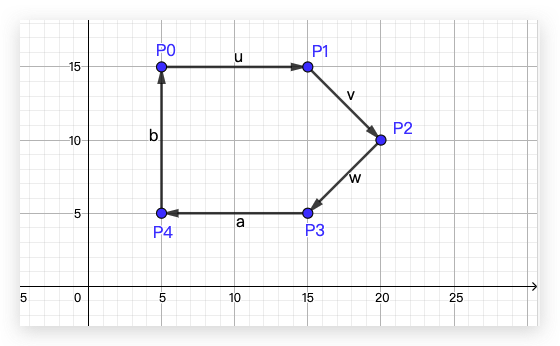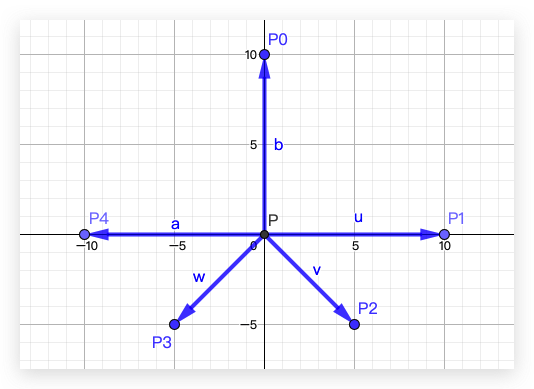### 3. 凸多边形边向量叉积均同号

$$\vec{u} \times \vec{v} < 0 \ \vec{v} \times \vec{w} < 0 \ \vec{w} \times \vec{a} < 0 \ \vec{a} \times \vec{b} < 0 \$$

### 4. 凹多边形边向量叉积不同号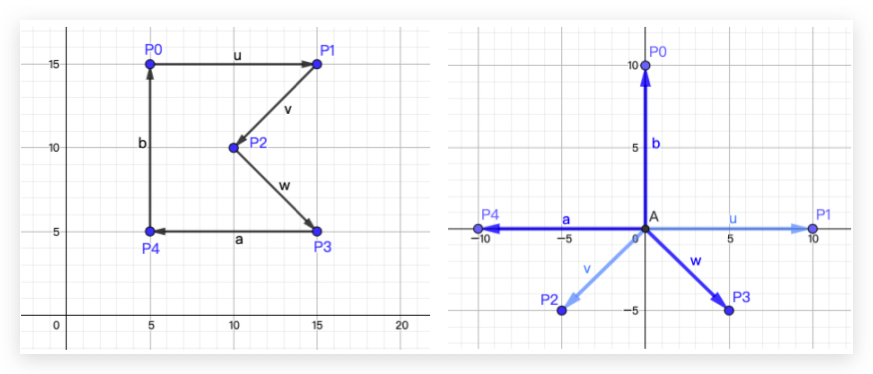$$\vec{u} \times \vec{v} < 0 \ \vec{v} \times \vec{w} > 0 \ \vec{w} \times \vec{a} < 0 \ \vec{a} \times \vec{b} < 0 \$$

### 5. 代码实现判别凹多边形